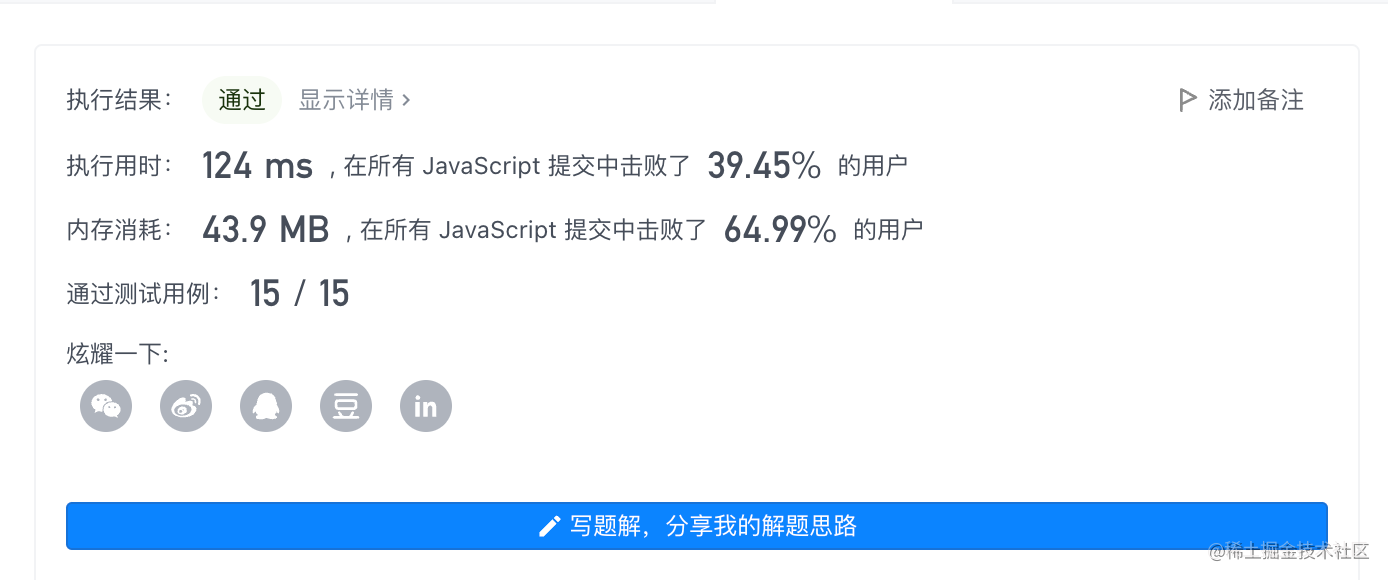2022-11-1700

1. 题目
2. 解

# 1. 题目

• `NumArray(int[] nums)` 使用数组 nums 初始化对象
• `int sumRange(int i, int j)` 返回数组 nums 从索引 `i` 到 `j（i ≤ j）` 范围内元素的总和，包含 `i``j` 两点（也就是 `sum(nums[i], nums[i + 1], ... , nums[j])`）

``````输入：
["NumArray", "sumRange", "sumRange", "sumRange"]
[[[-2, 0, 3, -5, 2, -1]], [0, 2], [2, 5], [0, 5]]

[null, 1, -1, -3]

NumArray numArray = new NumArray([-2, 0, 3, -5, 2, -1]);
numArray.sumRange(0, 2); // return 1 ((-2) + 0 + 3)
numArray.sumRange(2, 5); // return -1 (3 + (-5) + 2 + (-1))
numArray.sumRange(0, 5); // return -3 ((-2) + 0 + 3 + (-5) + 2 + (-1))
``````

• 0 <= nums.length <= 104
• -105 <= nums[i] <= 105
• 0 <= i <= j < nums.length
• 最多调用 104 次 sumRange 方法

# 2. 解

```javascript```/**
* @param {number[]} nums
*/
var NumArray = function(nums) {
const len = nums.length
this.nums = new Array(len).fill(0)
for(let i = 0; i< len; i++) {
this.nums[i + 1] = this.nums[i] + nums[i]
}
};

/**
* @param {number} left
* @param {number} right
* @return {number}
*/
NumArray.prototype.sumRange = function(left, right) {
return this.nums[right + 1] - this.nums[left]
};
``````• 时间复杂度：初始化 O(n)，每次检索 O(1)
• 空间复杂度： O(n)